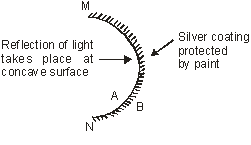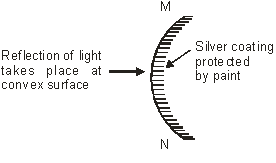# 10th Physics | Light | Spherical Mirrors

## SPHERICAL MIRRORS

A mirror whose reflecting surface is a part of a hollow sphere of glass is known as a spherical mirror.

Spherical mirrors are of two types :

### CONCAVE MIRROR

A concave mirror is that spherical mirror in which the reflecting surface is towards the centre of the sphere (i.e. inside the mirror) of which the mirror is a part. i.e. the reflection of light takes place at the concave surface (or bent-in-surface). As a concave mirror converges the parallel beam of light falling on it, therefore, it is also called a converging mirror. [figure 1]Concave mirror

Figure 1

### CONVEX MIRROR

A convex mirror is that spherical mirror in which the reflecting surface is away from the centre of the sphere (i.e. bulging out) of which mirror is a part i.e. reflection of light occurs at convex surface or the bulging out surface. As a convex mirror diverges the parallel beam of light falling on it, therefore it is also called a diverging mirror. [figure 2]Convex mirror

Figure 2

This site uses Akismet to reduce spam. Learn how your comment data is processed.